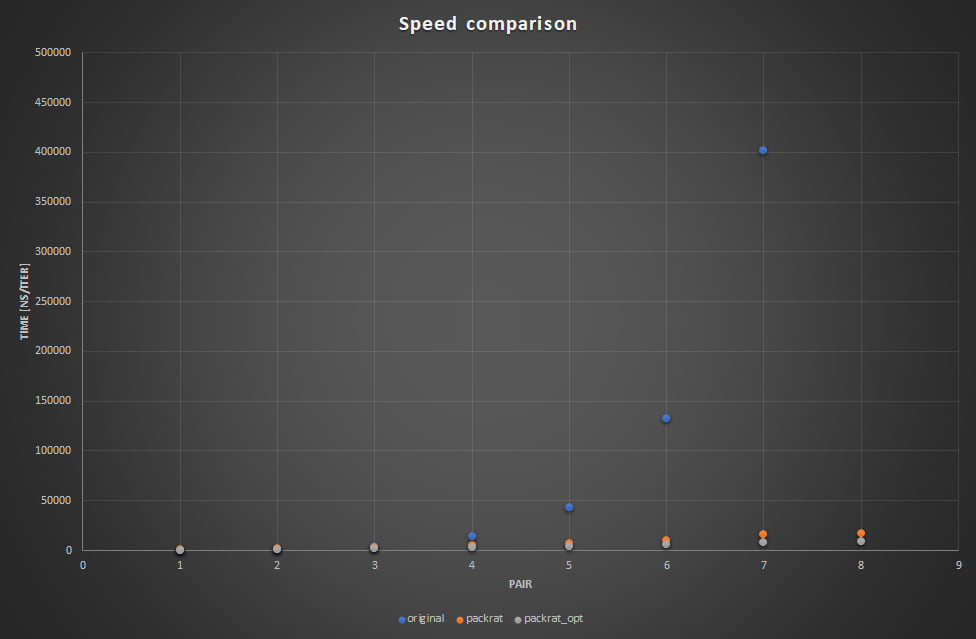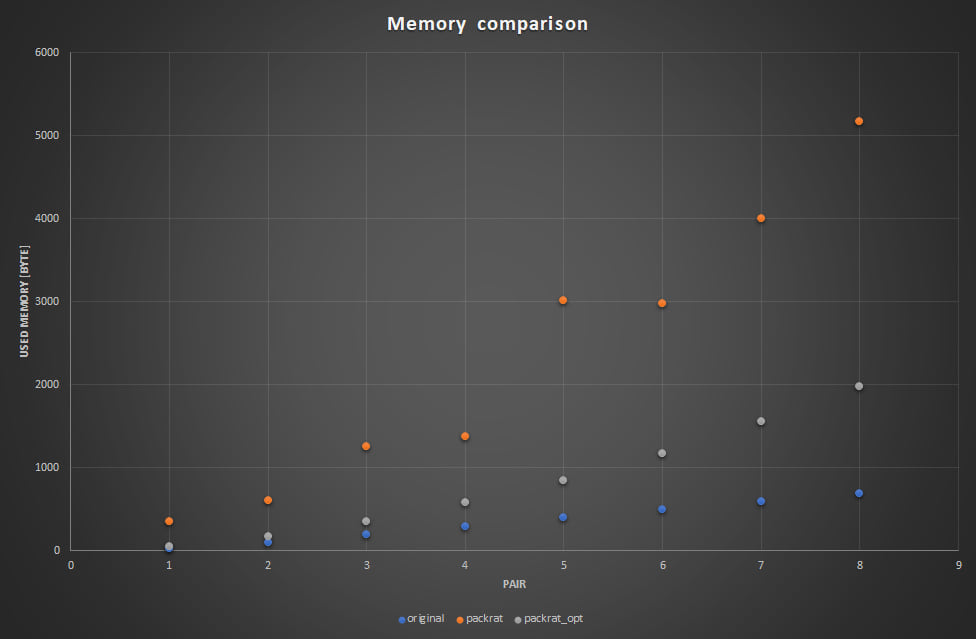## nom-packrat

Extension of nom to apply Packrat Parsing

### 16 releases

 0.7.0 Mar 23, 2023 Aug 31, 2021 Nov 26, 2020 Apr 1, 2020 Jul 28, 2019

#54 in Parser tooling

Used in 11 crates (2 directly)

MIT/Apache

10KB
122 lines

# nom-packrat

Extension of nom to apply "Packrat Parsing".

## Requirement

nom must be 5.0.0 or later. nom-packrat can be applied to function-style parser only.

## Usage

``````[dependencies]
nom-packrat = "0.7.0"
``````

## Example

``````use nom::character::complete::char;
use nom::IResult;
use nom_packrat::{init, packrat_parser, storage};

// Declare storage used by packrat_parser
storage!(String);

// Apply packrat_parser by custom attribute
#[packrat_parser]
pub fn parser(s: &str) -> IResult<&str, String> {
let (s, x) = char('a')(s)?;
Ok((s, x.to_string()))
}

fn main() {
let input = "a";

// Initialize before parsing
init!();
let result = parser(input);

println!("{:?}", result);
}
``````

## Performance

### Syntax

``````<S> ::= <T> + <S> | <T> - <S> | <T>
<T> ::= ( <S> ) | a
``````

### Input

The following 8 patterns. The first pattern is named as "pair 0" and the last is "pair 7".

``````a
(a)
((a))
(((a)))
((((a))))
(((((a)))))
((((((a))))))
(((((((a)))))))
``````

### Result

• original : the original nom parser
• packrat : all parsers with `#[packrat_parser]`
• packrat_opt: only `<T>` with `#[packrat_parser]`

This is an edge case. The execution time of the original parser increases exponentially. By packrat pasring, the time becomes linear. Instead packrat parsers consume more memory than the original parser.